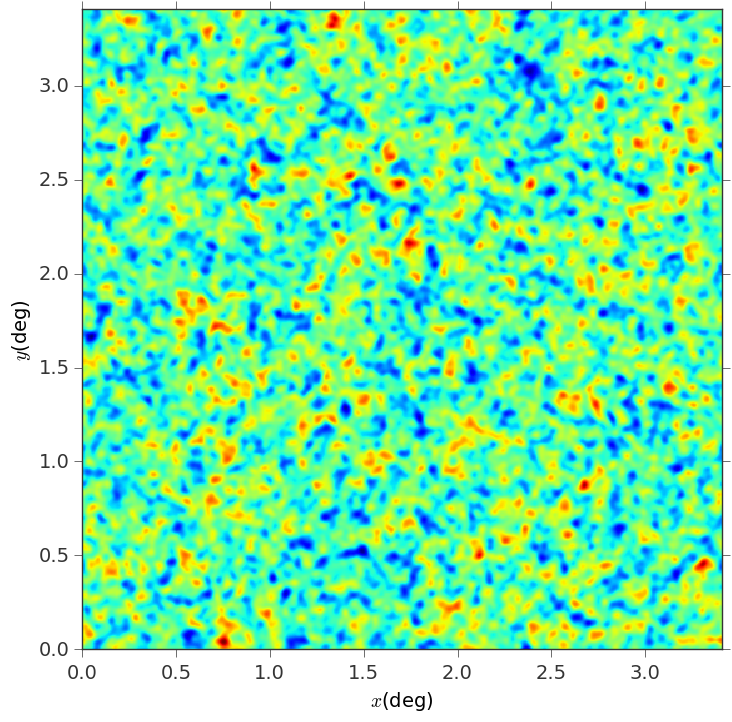# Generate a realization of a gaussian random field with known power spectrum¶

"""
Generate gaussian random fields with a known power spectrum

"""

import numpy as np
import matplotlib.pyplot as plt

from astropy.units import deg

from lenstools import GaussianNoiseGenerator

#Set map side angle, and number of pixels on a side
num_pixel_side = 512
side_angle = 3.41 * deg

#Read the power spectrum (l,Pl) from an external file, and load it in numpy array format (the generator interpolates the power spectrum between bins)

#Instantiate the gaussian noise generator
gen = GaussianNoiseGenerator(shape=(num_pixel_side,num_pixel_side),side_angle=side_angle,label="convergence")

#Generate one random realization
gaussian_map = gen.fromConvPower(np.array([l,Pl]),seed=1,kind="linear",bounds_error=False,fill_value=0.0)

#gaussian_map is a ConvergenceMap instance
gaussian_map.visualize()
gaussian_map.savefig("example_map.png")


This is the result